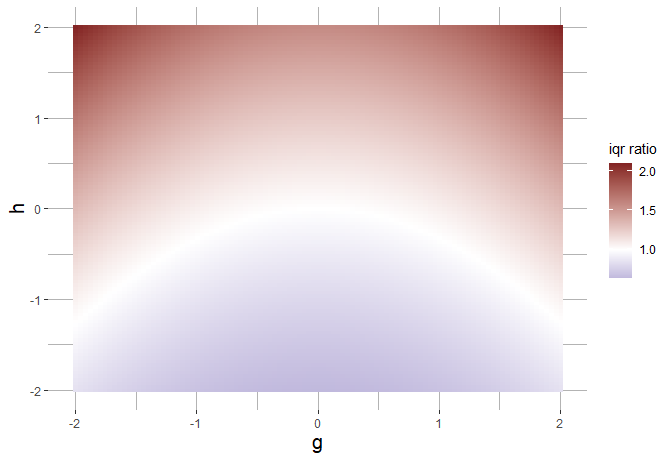# Replication of figures from the q-q boxplot paper

This vignette replicates the figures found in “The q-q boxplot” (citation coming soon).

First load the ‘qqboxplot’ package and the relevant packages from the ‘tidyverse’.

library(dplyr)
library(ggplot2)
library(qqboxplot)

## Figure 1

Figure 1 is meant to be a quick comparison of boxplots, q-q plots, and q-q boxplots. It uses a random sample of genes from one autism and one control patient and determines if the log of the gene expression counts can be modeled as lognormal.

#set value for text size so consistent across figures (only applies to figure 1)
eltext <- 12

#q-q boxplot
qqbox <- expression_data %>%
ggplot(aes(specimen, log_count)) + geom_qqboxplot(varwidth = TRUE, notch = TRUE) +
ylab('logged normalized expression') + ggtitle("c) q-q boxplot") +
theme(plot.title=element_text(size=eltext, face="plain", hjust=0.5), axis.title.x = element_text(size=eltext), axis.title.y = element_text(size=eltext),
panel.border = element_blank(), panel.background = element_rect(fill="white"),
panel.grid.major = element_line(colour = "grey70"),
panel.grid.minor = element_line(colour="grey80"))

# regular boxplot
box <- expression_data %>%
ggplot(aes(specimen, log_count)) + geom_boxplot(varwidth = TRUE, notch = TRUE) +
ylab('logged normalized expression') + ggtitle('a) boxplot') +
theme(plot.title=element_text(size=eltext, face="plain", hjust=0.5), axis.title.x = element_text(size=eltext), axis.title.y = element_text(size=eltext),
panel.border = element_blank(), panel.background = element_rect(fill="white"),
panel.grid.major = element_line(colour = "grey70"),
panel.grid.minor = element_line(colour="grey80"))

override.shape <- c(16, 17)
#q-q plot
qq <- expression_data %>%
ggplot(aes(sample=log_count)) + geom_qq(aes(color=specimen, shape=specimen)) +
xlab('theoretical normal distribution') +
ylab('logged normalized expression') + ggtitle('b) q-q plot') +
labs(color="specimen") +
guides(color = guide_legend(override.aes = list(shape=override.shape)), shape=FALSE) +
theme(plot.title=element_text(size=eltext, face="plain", hjust=0.5), axis.title.x = element_text(size=eltext), axis.title.y = element_text(size=eltext),
panel.border = element_blank(), panel.background = element_rect(fill="white"),
panel.grid.major = element_line(colour = "grey70"),
panel.grid.minor = element_line(colour="grey80"),
legend.position = c(0.8, 0.2))
#> Warning: guides(<scale> = FALSE) is deprecated. Please use guides(<scale> =
library(gridExtra)
#>
#> Attaching package: 'gridExtra'
#> The following object is masked from 'package:dplyr':
#>
#>     combine
# combine the plots
gridExtra::grid.arrange(box, qq, qqbox, ncol=3)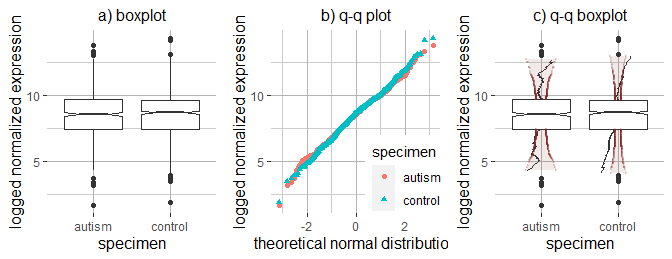## Figure 2

Figure 2 in the paper shows boxplots for simulated t-distributions (and one simulated normal distribution with mean=2). simulated_data contains two columns, “y” and “group”.
“group” specifies the distribution the data (“y”) comes from.

simulated_data %>%
ggplot(aes(factor(group, levels=c("normal, mean=2", "t distribution, df=32", "t distribution, df=16", "t distribution, df=8", "t distribution, df=4")), y=y)) +
geom_boxplot(notch=TRUE, varwidth = TRUE) +
xlab(NULL) +
ylab(NULL) +
theme(axis.text.x = element_text(angle = 23, size = 15), axis.title.y = element_text(size=15),
panel.border = element_blank(), panel.background = element_rect(fill="white"),
panel.grid = element_line(colour = "grey70"))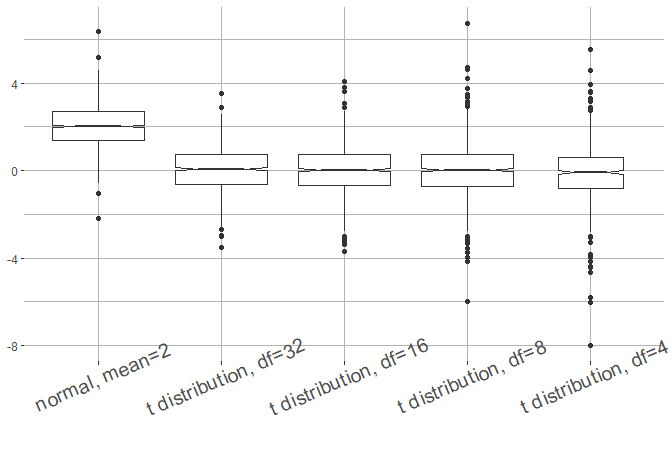## Figure 3

Figure 3 is the same data, visualized with a q-q plot compared with the theoretical normal distribution.

override.shape <- c(16, 17, 15, 3, 7)

simulated_data %>% ggplot(aes(sample=y, color=factor(group, levels=c("normal, mean=2", "t distribution, df=32", "t distribution, df=16", "t distribution, df=8", "t distribution, df=4")),
shape=factor(group, levels=c("normal, mean=2", "t distribution, df=32", "t distribution, df=16", "t distribution, df=8", "t distribution, df=4")))) +
geom_qq() + geom_qq_line() + labs(color="distribution") +
xlab("Normal Distribution") +
ylab("Simulated Datasets") +
guides(color = guide_legend(override.aes = list(shape=override.shape)), shape=FALSE) +
theme(axis.title.x = element_text(size=15), axis.title.y = element_text(size=15),
panel.border = element_blank(), panel.background = element_rect(fill="white"),
panel.grid = element_line(colour = "grey70"))
#> Warning: guides(<scale> = FALSE) is deprecated. Please use guides(<scale> =
#> "none") instead.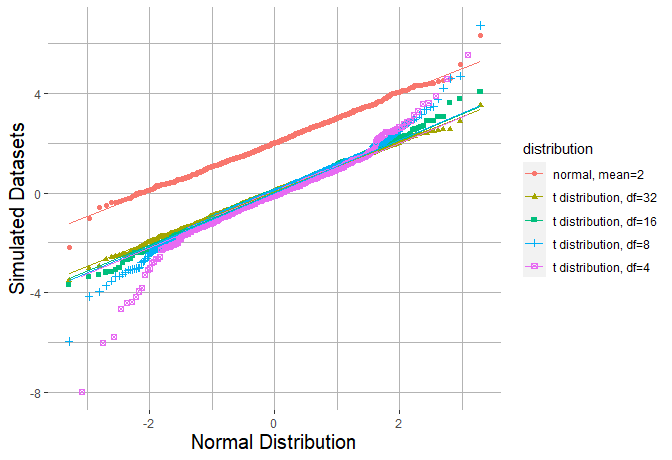## Figure 4

Figure 4 is the same data, visualized with q-q boxplots, compared with the theoretical normal distribution. Note in this figure that reference_dist = “norm” is chosen to specify that the normal distribution should be the reference distribution.

simulated_data %>%
ggplot(aes(factor(group, levels=c("normal, mean=2", "t distribution, df=32", "t distribution, df=16", "t distribution, df=8", "t distribution, df=4")), y=y)) +
geom_qqboxplot(notch=TRUE, varwidth = TRUE, reference_dist="norm") +
xlab("reference: normal distribution") +
ylab(NULL) +
guides(color=FALSE) +
theme(axis.text.x = element_text(angle = 23, size = 15), axis.title.y = element_text(size=15),
axis.title.x = element_text(size=15),
panel.border = element_blank(), panel.background = element_rect(fill="white"),
panel.grid = element_line(colour = "grey70"))
#> Warning: guides(<scale> = FALSE) is deprecated. Please use guides(<scale> =
#> "none") instead.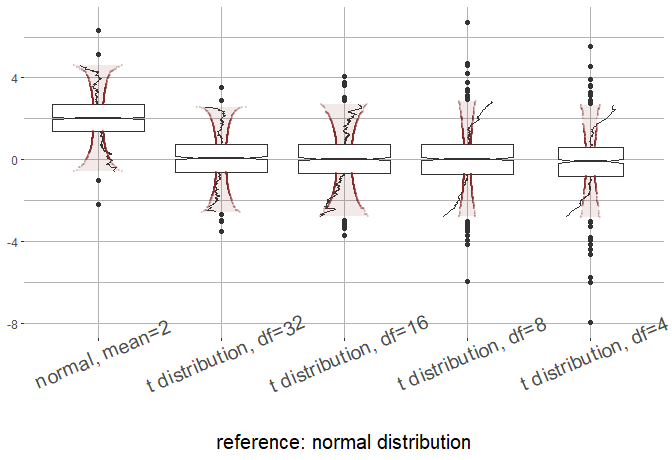simulated data was created by running the following code:

tibble(y=c(rnorm(1000, mean=2), rt(1000, 16), rt(500, 4),
rt(1000, 8), rt(1000, 32)),
group=c(rep("normal, mean=2", 1000),
rep("t distribution, df=16", 1000),
rep("t distribution, df=4", 500),
rep("t distribution, df=8", 1000),
rep("t distribution, df=32", 1000)))

## Figure 5

Figure 5 shows the same data from Figure 4, but compared against a simulated normal distribution, with mean=5 and variance=1. Note that the reference dataset comparison_dataset is a separate vector and is not contained in the dataset simulated_data.

simulated_data %>%
ggplot(aes(factor(group, levels=c("normal, mean=2", "t distribution, df=32", "t distribution, df=16", "t distribution, df=8", "t distribution, df=4")), y=y)) +
geom_qqboxplot(notch=TRUE, varwidth = TRUE, compdata=comparison_dataset) +
xlab("reference: simulated normal dataset") +
ylab(NULL) +
theme(axis.text.x = element_text(angle = 23, size = 15), axis.title.y = element_text(size=15),
axis.title.x = element_text(size=15),
panel.border = element_blank(), panel.background = element_rect(fill="white"),
panel.grid = element_line(colour = "grey70"))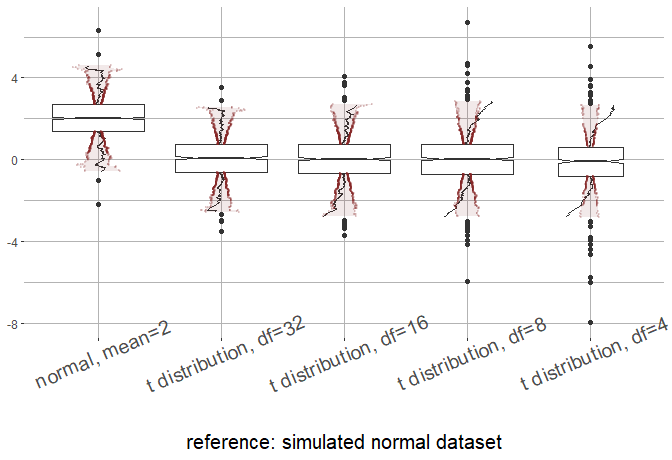The vector comparison_dataset was simulated as follows

rnorm(1000, 5)

## Figure 6

Figure 6 uses dataset “indicators” from this package. Male, 2008 serves as a base case. Note that the deviation whiskers for Male 2008 are straight since it is being compared with itself.

comparison_data <- indicators %>% filter(year==2008 & Series Code=="SL.TLF.ACTI.1524.MA.NE.ZS")

indicators %>%
#change the labels in series name to shorter titles
mutate(Series Name= ifelse(
Series Name=="Labor force participation rate for ages 15-24, male (%) (national estimate)",
"Male ages 15-24",
"Female ages 15-24")) %>%
ggplot(aes(as.factor(year), y=indicator))+
geom_qqboxplot(notch=TRUE, varwidth = TRUE, compdata=comparison_data\$indicator) +
xlab("Year") +
ylab("Country level labor force\nparticipation rate (%)") +
facet_wrap(~factor(Series Name, levels = c("Male ages 15-24", "Female ages 15-24"))) +
theme(strip.text = element_text(size=12), axis.text.x = element_text(size = 15), axis.title.x = element_text(size=15),
axis.title.y = element_text(size=12),
panel.border = element_blank(), panel.background = element_rect(fill="white"),
panel.grid = element_line(colour = "grey70"))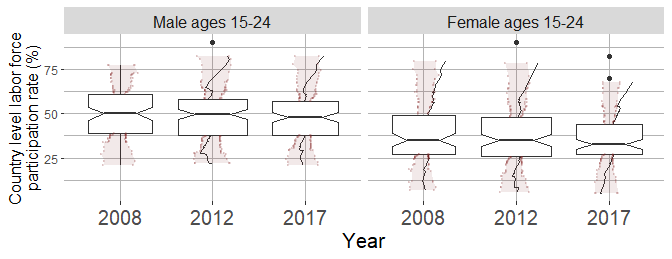## Figure 7

Figure 7 is the same data visualized with a violin plot.

indicators %>%
#change the labels in series name to shorter titles
mutate(Series Name= ifelse(
Series Name=="Labor force participation rate for ages 15-24, male (%) (national estimate)",
"Male ages 15-24",
"Female ages 15-24")) %>%
ggplot()+
geom_violin(aes(x=factor(year),y=indicator),fill='grey',trim=F, draw_quantiles = c(.25, .5, .75))+
geom_segment(aes(
x=match(factor(year),levels(factor(year)))-0.1,
xend=match(factor(year),levels(factor(year)))+0.1,
y=indicator,yend=indicator),
col='black'
) +
xlab("Year") +
ylab("Country level labor force\nparticipation rate (%)") +
facet_wrap(~factor(Series Name, levels = c("Male ages 15-24", "Female ages 15-24"))) +
theme(strip.text = element_text(size=12), axis.text.x = element_text(size = 15), axis.title.x = element_text(size=15),
axis.title.y = element_text(size=12),
panel.border = element_blank(), panel.background = element_rect(fill="white"),
panel.grid = element_line(colour = "grey70"))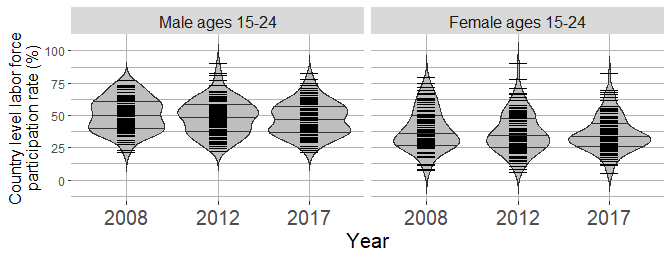## Figure 8

Figure 8 looks at spike counts from the V1 region of the brain under various grating orientations shown to a anesthetized macaque. The question her is if the spike counts can for each orientation can be reasonably modeled by a normal distribution (i.e. is the firing rate high enough to be reasonably approximated by a Gaussian)

spike_data %>% filter(region=="V1") %>%
ggplot(aes(factor(orientation), nspikes)) +
geom_qqboxplot(notch=TRUE, varwidth = TRUE, reference_dist="norm") +
xlab("orientation") +
ylab("spike count") +
theme(axis.text.x = element_text(size = 15), axis.text.y = element_text(size=14), axis.title.x = element_text(size=15),
axis.title.y = element_text(size=15),
panel.border = element_blank(), panel.background = element_rect(fill="white"),
panel.grid = element_line(colour = "grey70"))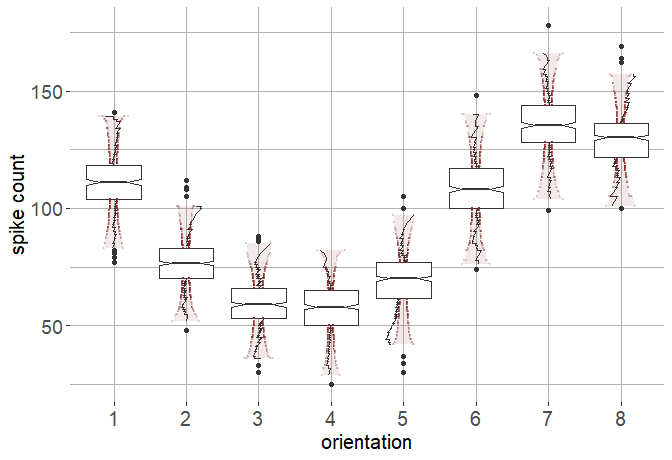## Figure 9

Same data, visualized with a bean plot.

spike_data %>% filter(region=="V1") %>%
ggplot()+
geom_violin(aes(x=factor(orientation),y=nspikes),fill='grey',trim=F, draw_quantiles = c(.25, .5, .75))+
geom_segment(aes(
x=match(factor(orientation),levels(factor(orientation)))-0.1,
xend=match(factor(orientation),levels(factor(orientation)))+0.1,
y=nspikes,yend=nspikes),
col='black'
) +
xlab("orientation") +
ylab("spike count") +
theme(axis.text.x = element_text(size = 15), axis.text.y = element_text(size=14), axis.title.x = element_text(size=15),
axis.title.y = element_text(size=15),
panel.border = element_blank(), panel.background = element_rect(fill="white"),
panel.grid = element_line(colour = "grey70"))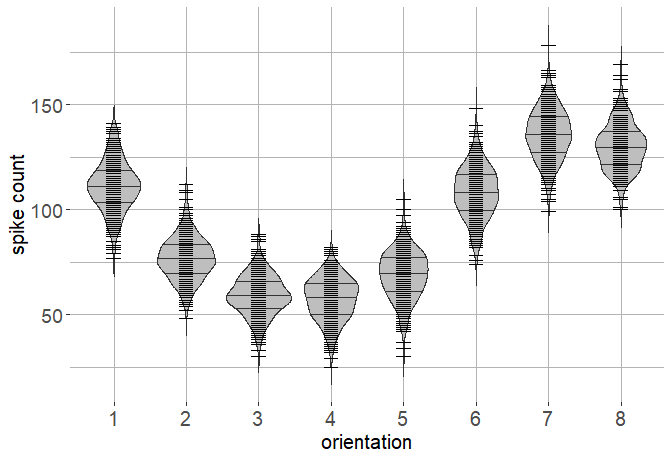## Figure 10

Figure 10 is the same setup but is looking at neurons in V2. Here we see that the firing rate is too low to be reasonably modeled as Gaussian.

spike_data %>% filter(region=="V2") %>%
ggplot(aes(factor(orientation), nspikes)) +
geom_qqboxplot(notch=TRUE, varwidth = TRUE, reference_dist="norm") +
xlab("orientation") +
ylab("spike count") +
theme(axis.text.x = element_text(size = 15), axis.text.y = element_text(size=14), axis.title.x = element_text(size=15),
axis.title.y = element_text(size=15),
panel.border = element_blank(), panel.background = element_rect(fill="white"),
panel.grid = element_line(colour = "grey70"))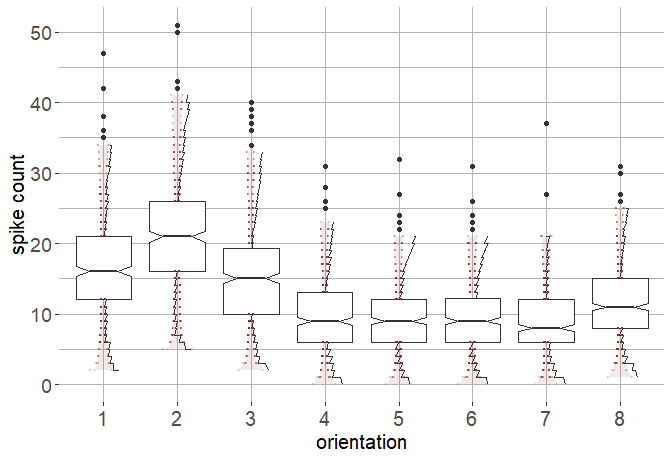## Figure 11

Same data as Figure 10, visualized with a bean plot.

spike_data %>% filter(region=="V2") %>%
ggplot()+
geom_violin(aes(x=factor(orientation),y=nspikes),fill='grey',trim=F, draw_quantiles = c(.25, .5, .75))+
geom_segment(aes(
x=match(factor(orientation),levels(factor(orientation)))-0.1,
xend=match(factor(orientation),levels(factor(orientation)))+0.1,
y=nspikes,yend=nspikes),
col='black'
) +
xlab("orientation") +
ylab("spike count") +
theme(axis.text.x = element_text(size = 15), axis.text.y = element_text(size=14), axis.title.x = element_text(size=15),
axis.title.y = element_text(size=15),
panel.border = element_blank(), panel.background = element_rect(fill="white"),
panel.grid = element_line(colour = "grey70"))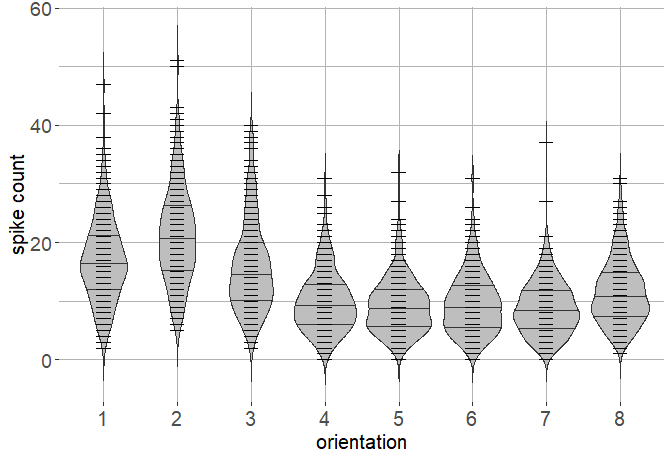## Figure 12

Figure 12 look at the firing rate for a population of neurons and asks if the population can be reasonably modeled as lognormal. It appears that, in general, this is not a reasonable distributional assumption.

population_brain_data %>%
ggplot(aes(x=ecephys_structure_acronym, y=log(rate))) +
geom_qqboxplot(notch=TRUE, varwidth = TRUE, reference_dist="norm") +
xlab("Brain regions") +
ylab("Log firing rate") +
facet_wrap(~fr_type) +
theme(axis.text.x = element_text(size = 15), axis.text.y = element_text(size=14),
axis.title.x = element_text(size=15)
, axis.title.y = element_text(size=15),
panel.border = element_blank(), panel.background = element_rect(fill="white"),
panel.grid = element_line(colour = "grey70"),
strip.text.x = element_text(size = 14))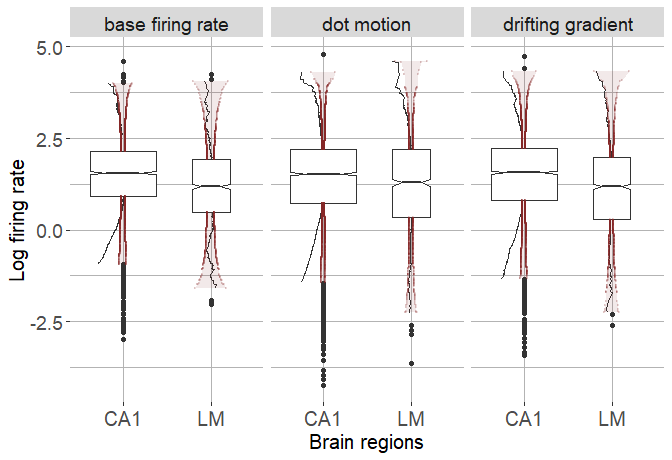## Figure 13

Same data as Figure 12, visualized with a violin plot.

population_brain_data %>%
ggplot(aes(x=ecephys_structure_acronym, y=log(rate))) +
geom_violin(fill='grey',trim=F, draw_quantiles = c(.25, .5, .75))+
xlab("Brain regions") +
ylab("Log firing rate") +
facet_wrap(~fr_type) +
theme(axis.text.x = element_text(size = 15), axis.text.y = element_text(size=14),
axis.title.x = element_text(size=15)
, axis.title.y = element_text(size=15),
panel.border = element_blank(), panel.background = element_rect(fill="white"),
panel.grid = element_line(colour = "grey70"),
strip.text.x = element_text(size = 14))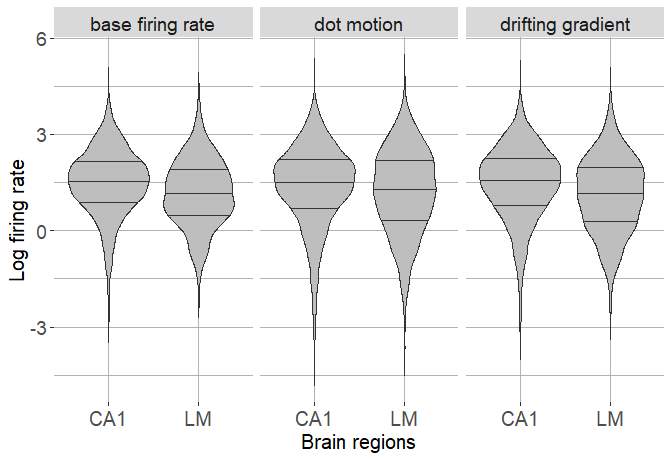## Appendix, Figure 14

library(scales)

# set grid
h_grid <- seq(from=-2, to=2, length.out = 101)
g_grid <- seq(from=-2, to=2, length.out = 101)
z_grid <- c(-.67448, .67448)

# transformation function
zfun <- function(h, g, z){
ifelse(g==0,
z*exp(h*z^2/2),
exp(h*z^2/2)*((exp(g*z)-1))/g)
}

# expand grid, calculate transformation of IQR.  Group the data by g and h values
# and then calculate the IQR for the transformed data and the IQR for the normal
# distribution (the second is the same for all groups, but is calculated here
# to avoid hardcoding).  Finally, compute the IQR ratio.
data <- as_tibble(expand.grid(h=h_grid, g=g_grid, z=z_grid)) %>%
mutate(zvals = zfun(h, g, z)) %>% group_by(h, g) %>%
summarize(iqr_mod = max(zvals) - min(zvals), iqr=max(z)-min(z)) %>%
ungroup() %>%
mutate(iqr_ratio = (iqr_mod) / iqr)
#> summarise() has grouped output by 'h'. You can override using the .groups argument.
data %>%
ggplot(aes(g, h)) + geom_raster(aes(fill=iqr_ratio)) + scale_fill_gradient2(low=muted("blue"), mid="white", high=muted("red"), midpoint=1) +
guides(fill=guide_colorbar(title="iqr ratio")) +
theme(axis.title.x = element_text(size=15), axis.title.y = element_text(size=15),
panel.border = element_blank(), panel.background = element_rect(fill="white"),
panel.grid = element_line(colour = "grey70"))